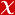## ON THE DIOPHANTINE EQUATION (5pn(2) - 1)(x)

HONAM MATHEMATICAL JOURNAL, vol.42, no.1, pp.139-150, 2020 (Journal Indexed in ESCI)• Publication Type: Article / Article
• Volume: 42 Issue: 1
• Publication Date: 2020
• Doi Number: 10.5831/hmj.2020.42.1.139
• Title of Journal : HONAM MATHEMATICAL JOURNAL
• Page Numbers: pp.139-150
• Keywords: Exponential Diophantine equation, Jacobi symbol, Baker's method, LINEAR-FORMS, 2 LOGARITHMS, CONJECTURE

#### Abstract

Let p be a prime number with p > 3, p 3 (mod 4) and let n be a positive integer. In this paper, we prove that the Diophantine equation (5pn(2) - 1)(x) + (p(p - 5)n(2) + 1)(y) = (pn)(z) has only the positive integer solution (x; y; z) = (1; 1; 2) where pn +/- 1 (mod 5). As an another result, we show that the Diophantine equation (35n(2) - 1)(x) + (14n(2) + 1)(y) = (7n)(z) has only the positive integer solution (x, y, z) = (1; 1; 2) where n +/- 3 (mod 5) or 5 vertical bar n. On the proofs, we use the properties of Jacobi symbol and Baker's method.